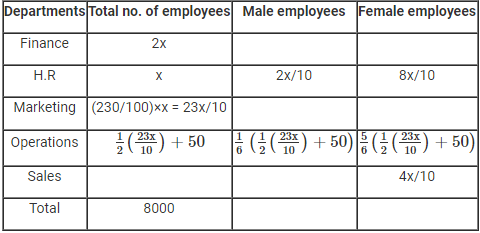Data Interpretation Quiz for SBI PO Mains

Directions (1 – 5): Answer the questions on the basis of the information given below.
In Mumbai branch of Bharat Heavy Electricals Ltd. There are 8000 employees working in five different departments – Finance, HR, Marketing, Operations and Sales. Also, each employee works in only one department. The number of employees in Finance is twice the number of employees in HR. The number of employees in Marketing is the highest and is 230% of the number of employees in HR. The number of male employees in the HR and Operations is the same and is half the number of female employees in Sales. The number of employees in Operations is 50 more than half the number of employees in Marketing. The ratio of the number of male employees to the number of female employees if HR is 1 : 4 and is Operations is 1 : 5.
1. What is the number of male employees in sales department?
A) 400
B) 1200
C) 1100
D) 550
E) None of these
2. What is the number of female employees in Finance department?
A) 200
B) 300
C) 1500
D) 500
E) Can’t be determined
3. What is the ratio of the number of male employees in Operations to the number of female employees in HR?
A) 1 : 5
B) 2 : 7
C) 1 : 4
D) 1 : 3
E) 2 : 5
4. Number of employees working in Finance constitutes what percentage of the total number of employees in the organization?
A) 30%
B) 46%
C) 25%
D) 15%
E) 8.75%
5. What is the total number of female employees in the organizations?
A) 1600
B) 2600
C) 5000
D) 3000
E) Can’t be determined
Directions (6 – 10): The following pie-chart shows the distribution of the total production of six factories and the table shows the percentage of packaged item in these factories and the ratio of defectives to non-defectives among these packaged productions.
 Factory %Packed Defective : Non-defective A 72 7 : 5 B 65 8 : 5 C 75 3 : 2 D 80 9 : 7 E 70 4 : 3 F 60 7 : 56. The packaged item production of Factory D is approximately what per cent of the packaged item production of Factory A ?
(a) 74%
(b) 70%
(c) 76%
(d) 84%
(e) 90 %
7. What is the difference between the total packaged production of Factory C and the total production of Factory E?
(a) 26500
(b) 15000
(c) 25500
(d) 24500
(e) 35500
8. What is the difference between the packaged defective production of factory B and the packaged non-defective production of Factory C ?
(a) 40500
(b) 30800
(c) 40600
(d) 40800
(e) 40900
9. The packaged non-defective production of Factory A is approximately what per cent of packaged defective production of factory E?
(a) 104%
(b) 103%
(c) 102%
(d) 105%
(e) 101%
10. The packaged defective production of Factory B is approximately what percentage more or less than its packaged non-defective production?
(a) 35%
(b) 40%
(c) 50%
(d) 55%
(e) 60%
Solutions:
(1 – 5):
Let total number of employees in H. R department be x and make table accordingly.1. C) Number of employees in Sales dept. = Total number of employees – no of employees in other dept.
= (8000 – 2000 – 1000 – 2300 - 1200) = 1500
Number of male employees in Sales dept. = 1500 – 400 = 1100
2. E) We can’t determine the number of female employees in Finance department as we don’t know the number of males in this department or ratio between male and female in this department.
3. C) Ratio of the number of male employees in Operations to the number of female employees in HR = 200 : 800 = 1 : 4
4. C)5. E) We can’t determine total number of female employees in the organizations because we don’t know the number of female employees in finance and marketing.
6. (a) required percentage = (14×80)/(21×72)×100=74% (approx)
7. (c) Packaged production of factory C = 8.5×16/100×75/100 lakh = 1.02 lakh
Total production of factory E = 8.5×15/100 = 1.275 lakh
Difference = 0.255 lakh = 25,500
8. (d) Packaged defective item production of factory B
=8.5×24/100×65/100×8/13 =0.816 lakh
Packaged non-defective item production of factory C
= 8.5×16/100×75/100×2/5=0.408 lakh.
Difference = 0.816 – 0.408 = 0.408 lakh = 40800
9. (d) Packaged non-defective item production of Factory A
= 8.5×21/100×72/100×5/12=0.5355 lakh
Packaged defective item production of factory E
= 8.5×15/100×70/100×4/7=0.51 lakh
Req.% = 0.5355/0.51×100 = 105%
10. (e) Req. % = (8-5)/5×100 = 60%